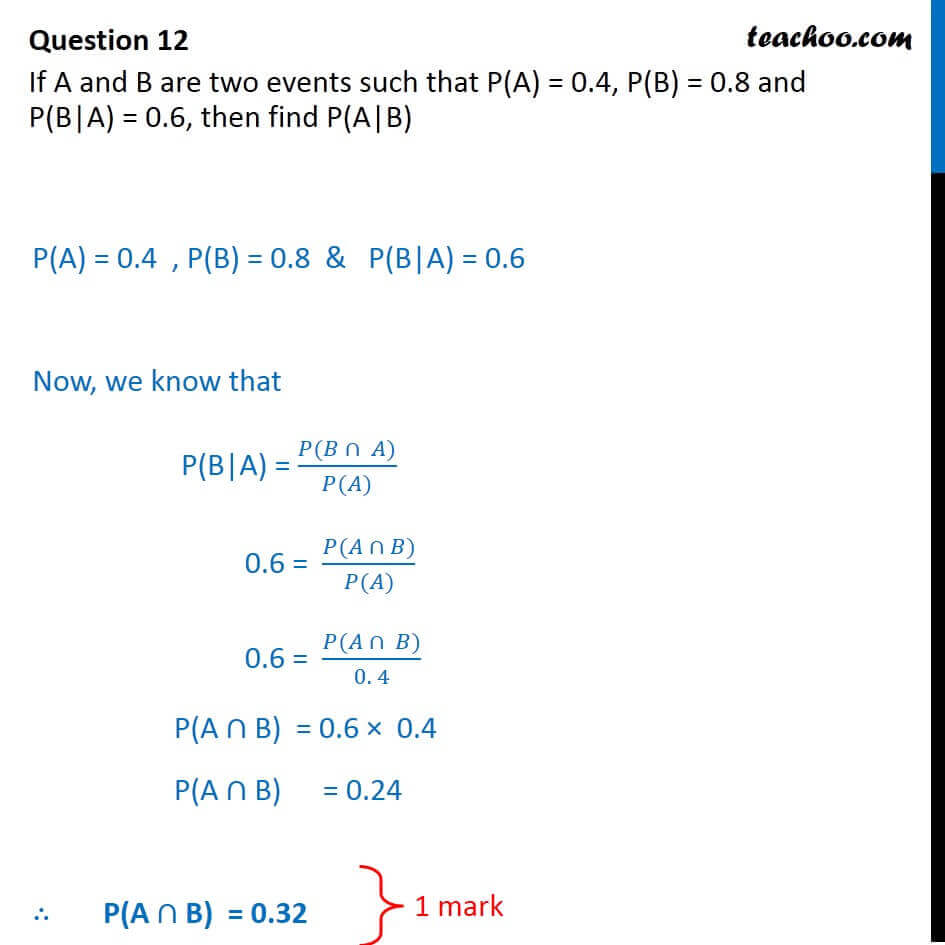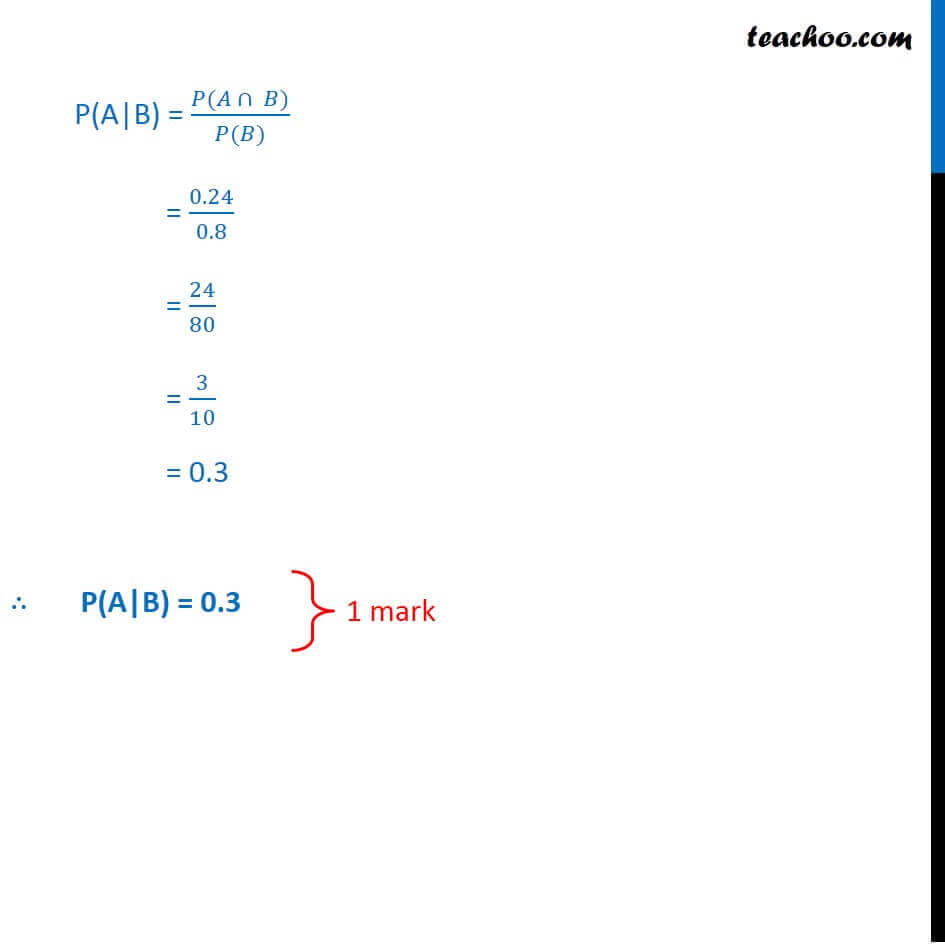CBSE Class 12 Sample Paper for 2018 Boards

Class 12
Solutions of Sample Papers and Past Year Papers - for Class 12 Boards

### If A and B are two events such that P(A) = 0.4, P(B) = 0.8 and P(B|A) = 0.6, then find P(A|B)

This is a question of CBSE Sample Paper - Class 12 - 2017/18.Learn in your speed, with individual attention - Teachoo Maths 1-on-1 Class

### Transcript

Question 12 If A and B are two events such that P(A) = 0.4, P(B) = 0.8 and P(B|A) = 0.6, then find P(A|B) P(A) = 0.4 , P(B) = 0.8 & P(B|A) = 0.6 Now, we know that P(B|A) = (𝑃(𝐵 ∩ 𝐴))/(𝑃(𝐴)) 0.6 = (𝑃(𝐴 ∩ 𝐵))/(𝑃(𝐴)) 0.6 = (𝑃(𝐴 ∩ 𝐵))/(0. 4) P(A ∩ B) = 0.6 × 0.4 P(A ∩ B) = 0.24 ∴ P(A ∩ B) = 0.32 P(A|B) = (𝑃(𝐴 ∩ 𝐵))/(𝑃(𝐵)) = 0.24/0.8 = 24/80 = 3/10 = 0.3 ∴ P(A|B) = 0.3